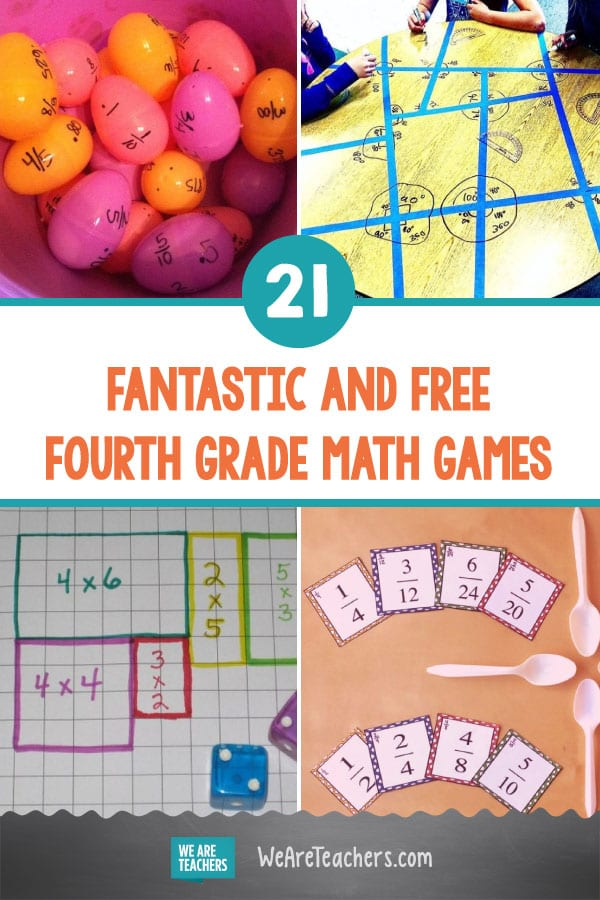# 21 Fantastic and Free Fourth Grade Math Games

These math games really up the fun factor!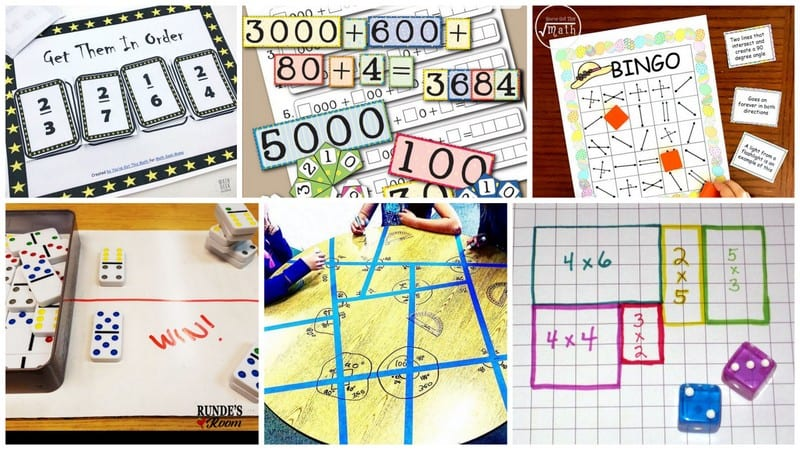Fourth grade math is full of new and important concepts. Fractions become more important, decimals show up for the first time, and division problems might have remainders! Make all the fourth grade common core math skills more fun with this roundup of free math games.

## 1. Make division fun with Remainders Wanted.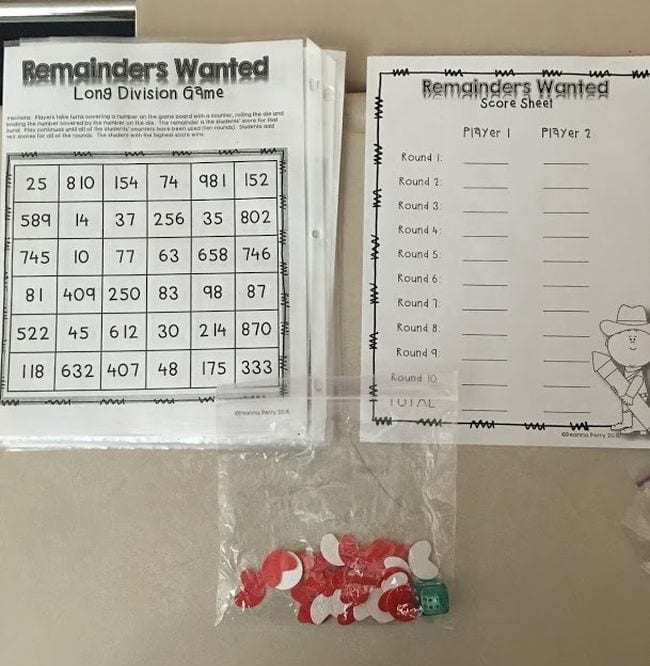Fourth grade math students learn to divide larger numbers, often with remainders. In this game, the remainder is the goal! For each turn, students place a counter over a number on the board, then roll the die to see what number they’ll be dividing it by. The remainder is their score for that turn!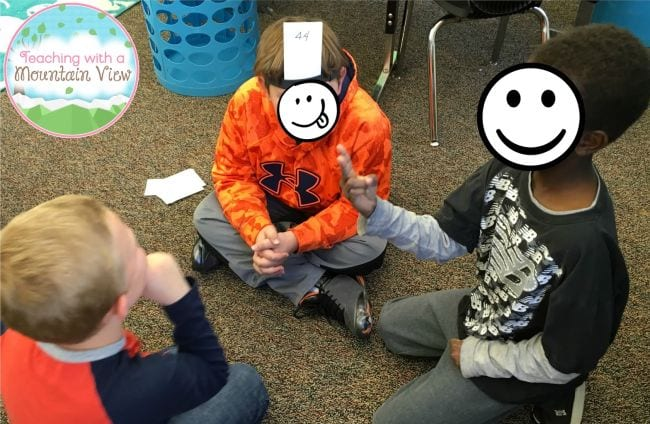Kids use dollar store headbands to hold cards showing multiplication products to their foreheads. Their partners try to get them to guess the number by telling them the factors, without saying the number itself.

## 3. Strategize to win the Factor Game.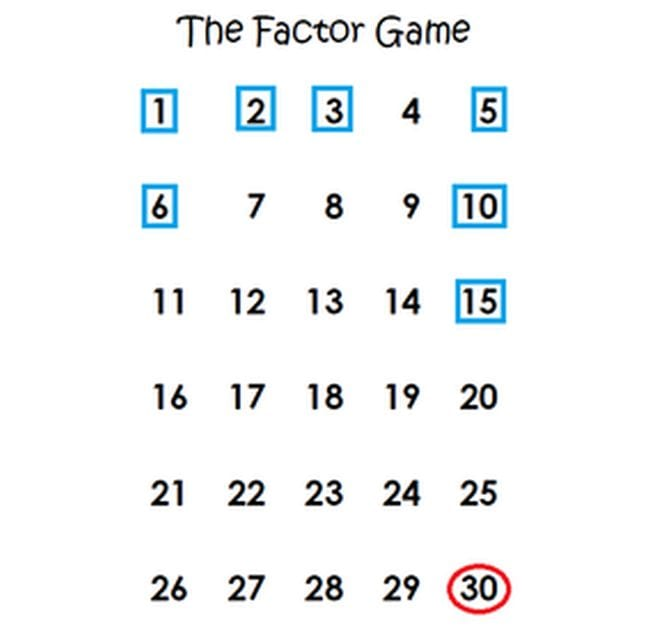Write the numbers from 1 to 30 as shown. The first player marks a number (their score for that round). The second player then marks all the remaining factors of that number (which add up to their score for that round). Play continues until all numbers are marked.

## 4. Introduce patterns by asking “What Do You Notice?”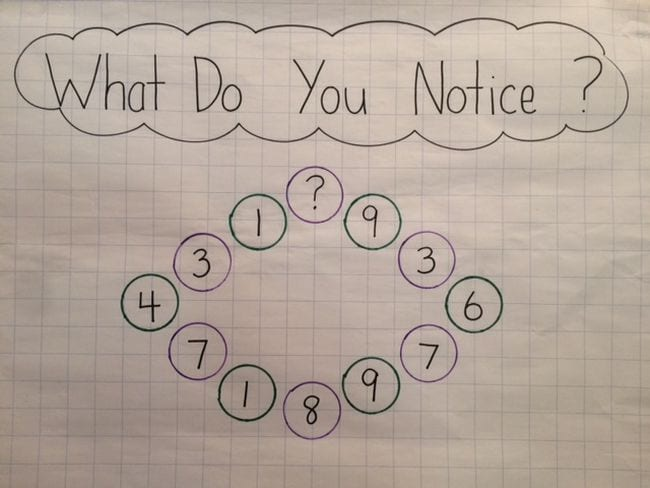Lay out a pattern problem and ask students to write down what they notice about it on sticky notes. Collect and talk about the notes to see which are helpful in finding the right number to fit the pattern. Award points to correct answers, if you like.

## 5. Guess then deduce with Number Pattern Solver.Draw a circle and set a number range, then tell fourth grade math students you have a pattern in mind. As they begin guessing numbers, write them inside the circle if they fit the pattern, and outside if they don’t. At first they’ll just be guessing, but eventually they’ll start to see a pattern emerge.

## 6. Roll to win Place Value Yahtzee.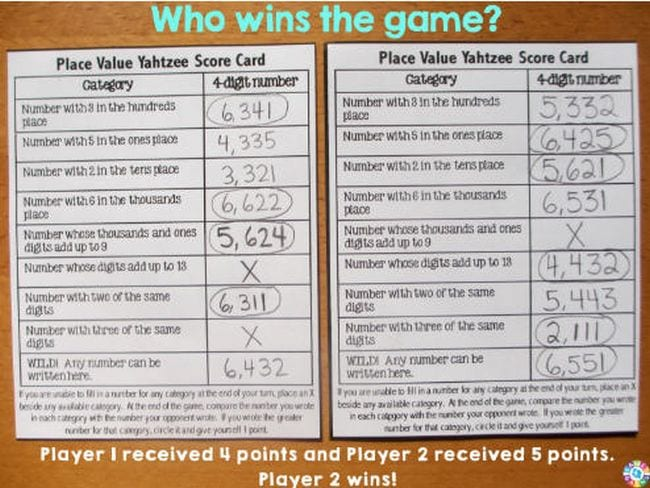These free printable Place Value Yahtzee boards include differentiated versions for various skill levels. Add some dice and you’re ready to play!

## 7. Use playing cards to practice decimal place values.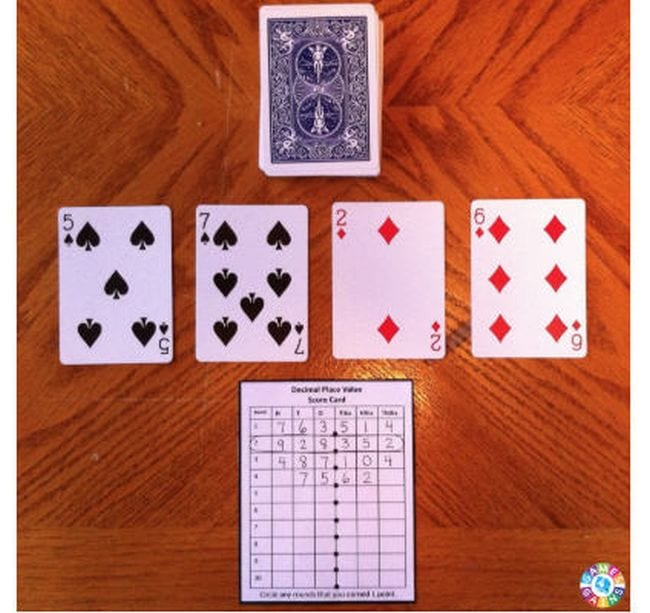Fourth grade math students take turns drawing cards, competing to build the highest possible number to the thousandths place.

## 8. Stack cards to learn expanded form.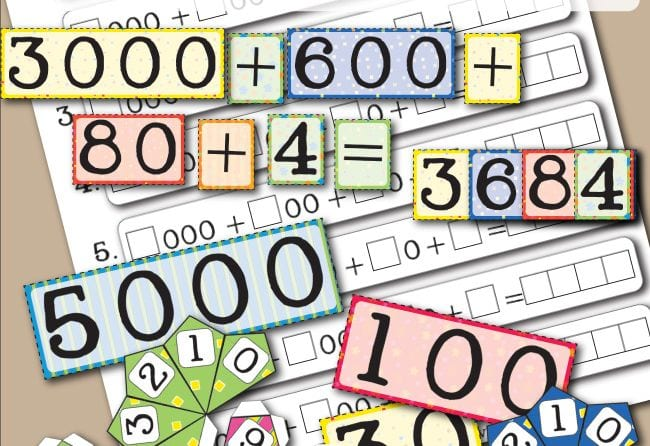Grab the free printable cards, then have kids roll the dice and choose a card that matches. They build the numbers on the included worksheet to get the total. For extra practice, have them write out the word names too.

## 9. Have a rounding practice snowball fight.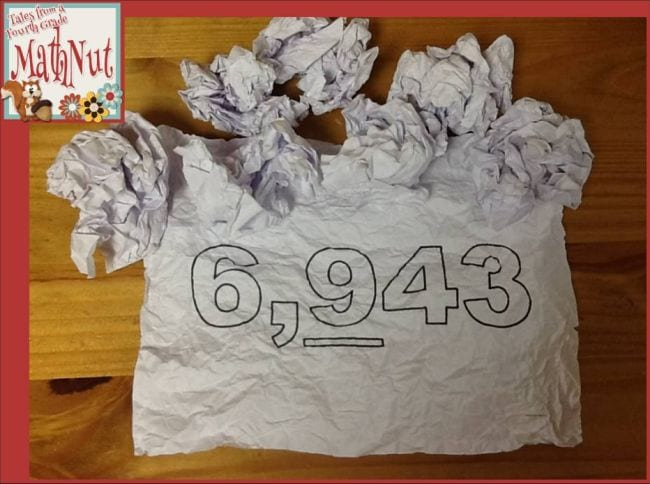Up for a little controlled chaos in the classroom? Write one number on each sheet of paper, underlining the place it should be rounded to. Pass out a few sheets per student, and have them crumple them up into “snowballs.” Allow a 30-second safe “snowball fight,” then have each student pick up a snowball and read the number out loud, rounding it correctly. Repeat!

## 10. Multiply large numbers to get Three In a Row.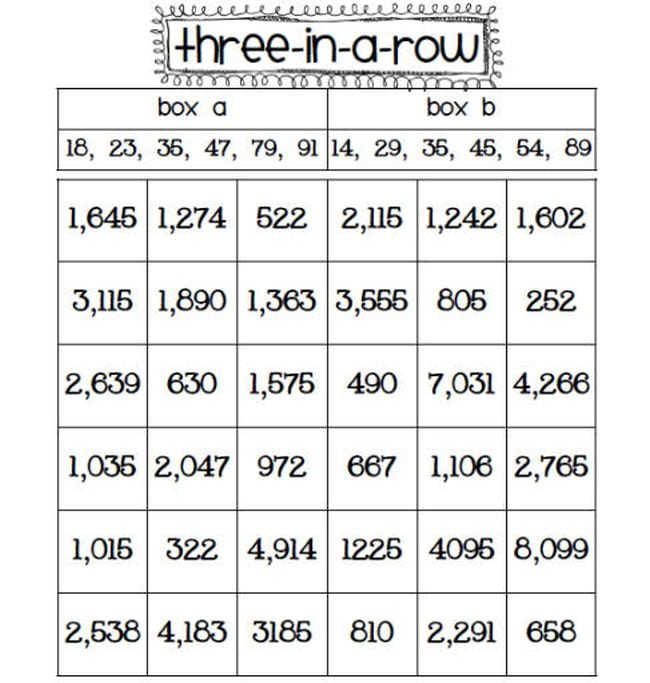Fourth grade math students work on multi-digit multiplication by choosing one number from box A and one from box B. They multiply them together, while their partner checks their answer on a calculator. If they get it right, they place a marker on the free printable board. The goal is to get three spots in a row. (Savvy players will use rounding to determine which numbers to pick next!)

## 11. Hold a Quotient Tournament.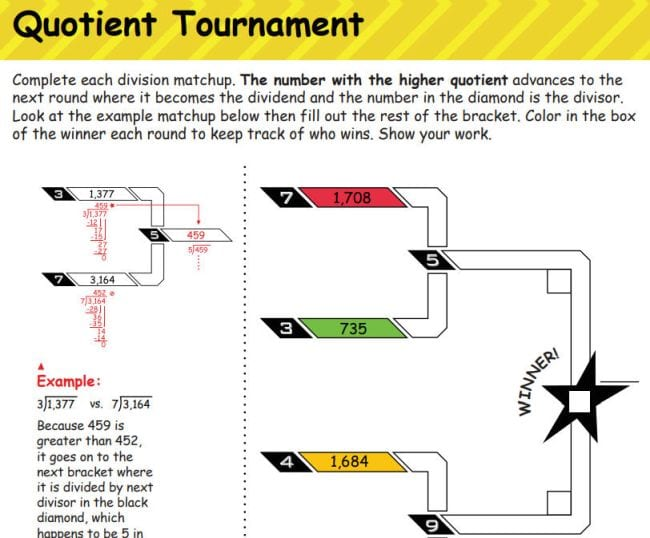Division problems go head-to-head to see which has the larger quotient. Students use the answer for each to complete the bracket for the next round.

## 12. Give Spoons an equivalent fraction twist.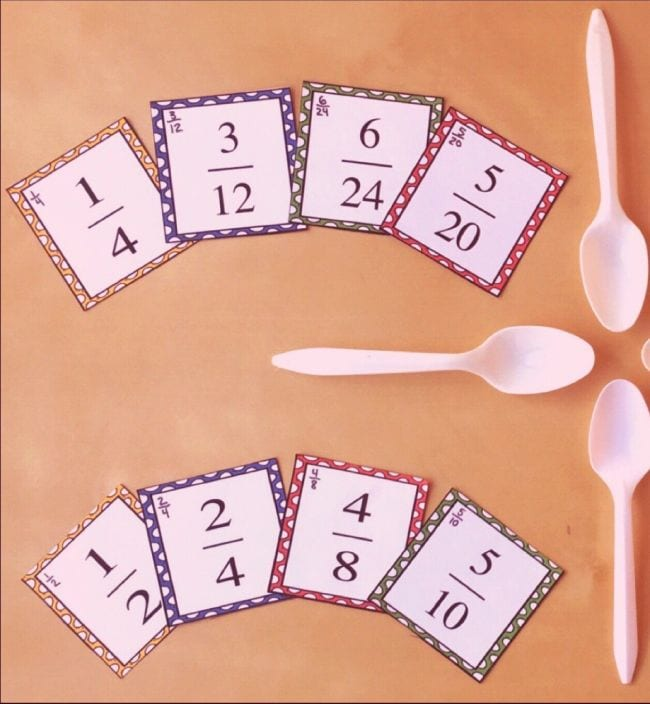Spoons is a classic and beloved card game where players race to match four-of-a-kind and grab a spoon in each round. In this version, they’re racing to match equivalent fractions (get some free printable cards to use at the link).

## 13. Get those fractions in order.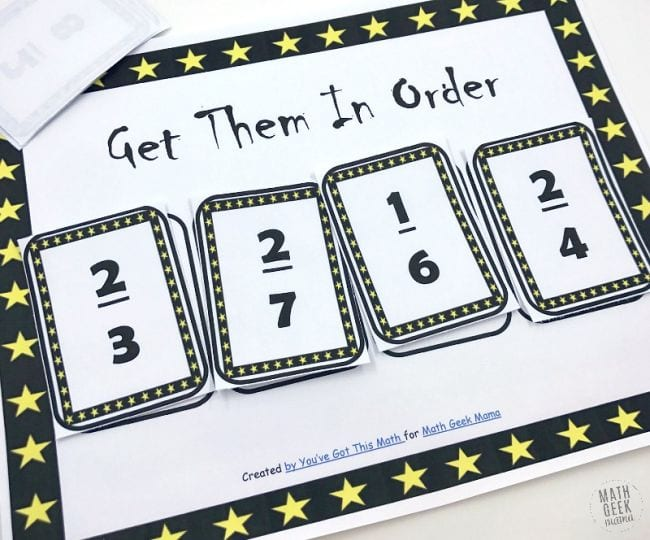Students draw fraction cards, swapping them out to build a series of four in ascending order. Learn how it works at the link.

## 14. Declare a fraction war with dominoes.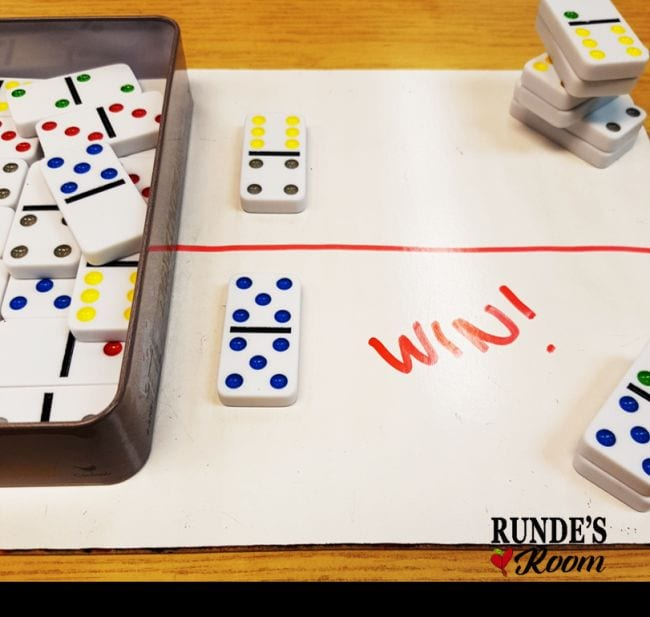Each student draws a domino and positions it as a fraction. Then they compare the two to see whose is larger. The winning student keeps both dominoes. (See more cool ways to use dominoes for fraction games at the link.)

## 15. Line kids up for decimal practice.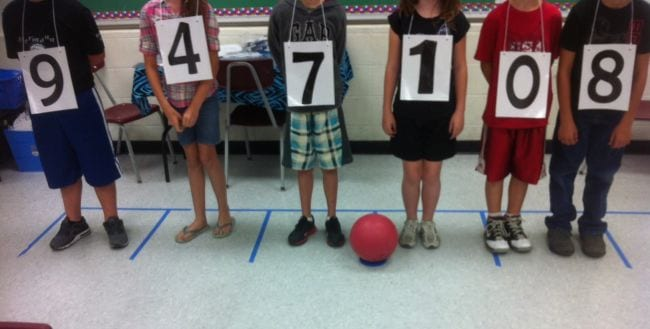Get fourth grade math students up and moving by assigning them numbers. Draw a six-digit number card, and have another student help them line up by saying things like, “The 9 is in the hundreds place.” Once they’re in line, ask some follow-up questions to confirm understanding. Learn more at the link.

## 16. Use plastic eggs to match up fractions and decimals.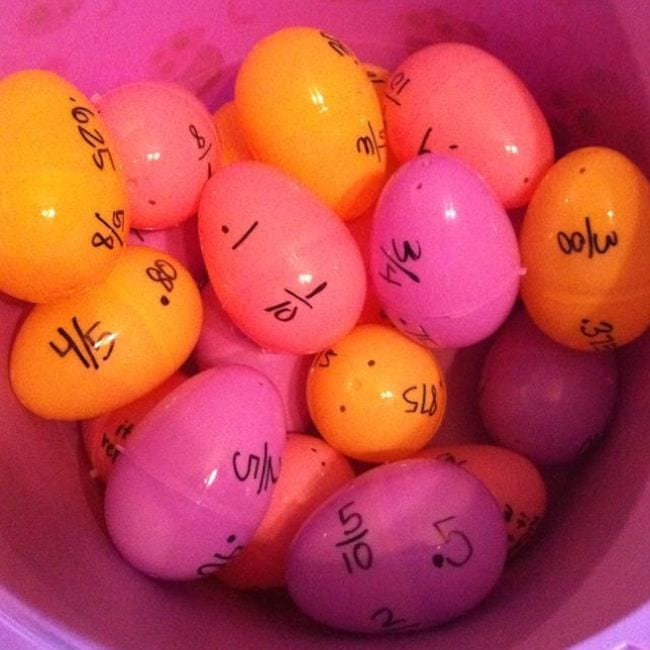Snag some plastic eggs from the dollar store and write fractions on one half, with equivalent decimals on the other. Kids work to match them up.

## 17. Battle with area and perimeter to fill a graph page.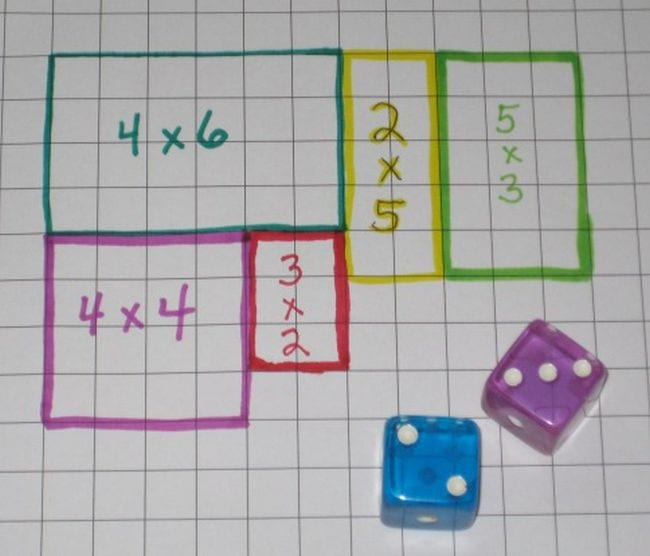Roll the dice to see the dimensions of your next rectangle, then mark it on the board. Try to fill your page completely before your partner does! (Have students write the area and perimeter in each block for practice along the way.)

## 18. Go on a measurement scavenger hunt.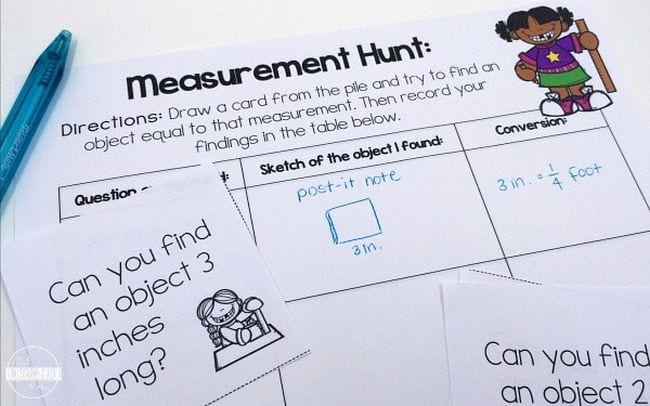Arm kids with measurement tools, then send them off to find objects that match the free printable challenge cards. Once they find it, they also need to convert the measurement within the same system (such as inches to feet).

## 19. Play geometry bingo.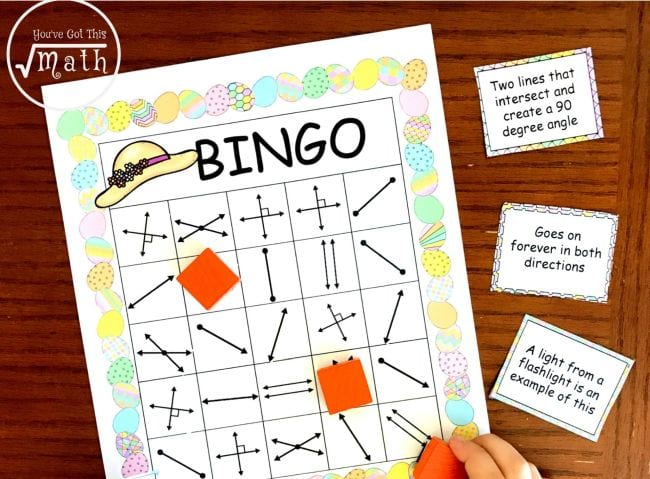Fourth grade math students are learning terms like line, ray, and types of angles. This free printable bingo game is a fun way to do it!

## 20. Tape off your tables for protractor practice.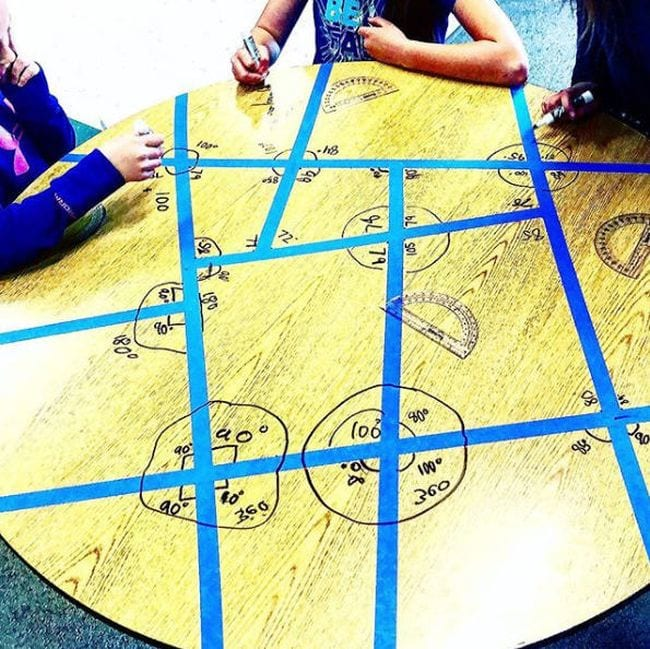Use dry erase markers and masking tape to give students lots of angles to explore and measure! If you can’t write on your tables, try using a big piece of butcher paper instead.

## 21. Fold shapes to discover symmetry.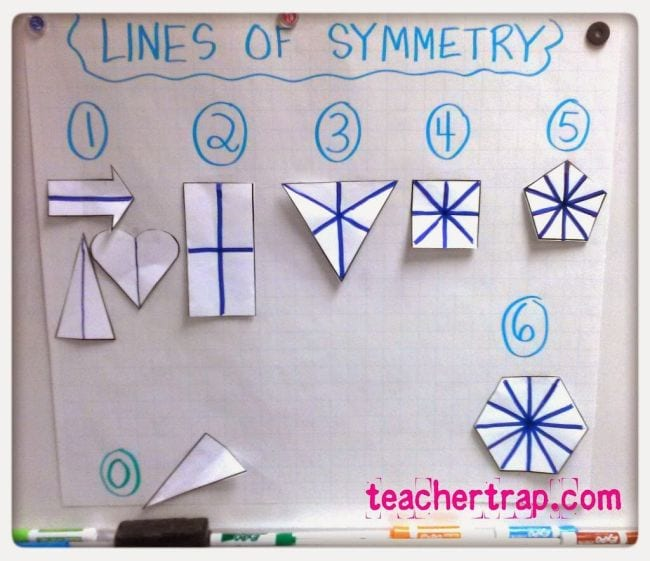Break students into groups and hand out a series of paper shapes. Challenge each group to experiment with folding their shapes to see which are symmetrical, and how many lines of symmetry they have.